# Covariance and correlation matrices

## A transcript of covariance and correlation matrices presentation, by Rebecca PillingerSo we're using multilevel modelling because we have dependent data. So for example if we have exam results, then exam results for pupils from the same school are likely to be more similar than exam results for pupils from different schools, or if we have heights, the heights of children in the same family are likely to be more similar than the heights of children in different families, and this is something that we saw in another audio recording, Measuring Dependency; we also saw that we can measure the dependency using something called rho, or the variance partitioning coefficient. But a question of interest is how the multilevel model actually takes into account this dependency: how does it cope with the fact that our exam results are more similar for pupils in the same school, or our heights are more similar for children from the same family? Well, in order to understand how it does this, we can take a look at the structure of the model using the correlation matrix, and to do that, first of all we'll look at the covariance matrix.

### Matrix 1So let's look first of all at the covariance matrix for a single-level model, so here we have a model of exam results for pupils within schools; the numbers along the top and down the left in red, green and blue are the numbers of the school, and just within those we have the numbers of the pupils, and then the entries of the matrix are the covariance[s] between each pair of pupils. So notice that it's not the covariance between the responses for each pair of pupils: what we're actually taking is the covariance of the response after we've controlled for the covariates, so we're basically subtracting the predicted value from the regression line from the response. Because we're interested in the dependency after we've controlled for the things we've put in the model.

So how do we work out what these covariances actually are? Well, we need to go back to the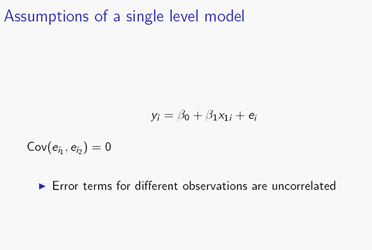assumptions of the single level model, and the one that chiefly concerns us here is this one, that the error terms for different observations are uncorrelated.So now, if we take the covariance of an observation with itself, so as we said we're subtracting from the response the predicted value (from the covariates), and we end up with the error terms, that's all that's left after we've taken the fixed part away. And this is just the covariance of the level one error term with itself- you can see we've got e_i1 and e_i1, the same thing, and when we have the covariance of the same thing, that's just the variance, so we've got the variance of e_i1, and we've defined that to be sigma squared_e, so for the same observation, the covariance is sigma squared_e so down the diagonal of this matrix [Matrix 1] we have sigma squared_eSo now, for two different observations, again we subtract the predicted value from the response, and we end up again with just the error terms. Now this covariance is the covariance between two different level one error terms, and we assumed that that was zero, so we just have zero.

And so all the off-diagonal terms in this matrix [Matrix 1] are zero. So now if we look at the correlation structure, we need to divide the covariance by the total variance to get the correlation; the total variance for a single level model is sigma squared_e, so all those diagonal terms are going to become 1 and the zeros will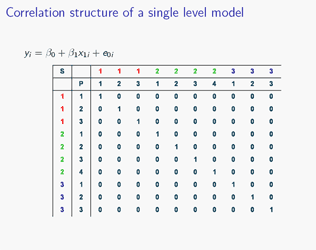stay zero. So now we can see that for the single level model, two different pupils are unrelated, whether they go to the same school, or whether they go to different schools.

### Matrix 2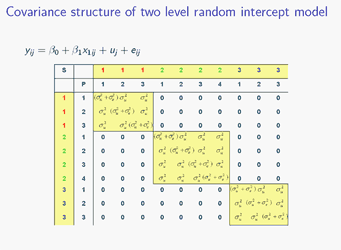So what happens with a multilevel model? Well, here we're fitting a two level random intercept model, and again we've put the covariance between each pair of pupils into the matrix, again we're taking the covariance after we've controlled for the covariates (the x variables) and again, in order to work out what we should be putting in this matrix, we need to look at the assumptions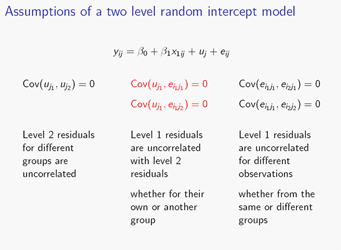of this model. So first of all we have the assumption that the level two error terms for two different groups are uncorrelated, and then we have an assumption that level one error terms for different observations are also uncorrelated - so that's true whether it's the level one error terms for two different observations from the same group, or the level one error terms for two different observations from different groups. And then finally we assume that the level one and level two error terms are uncorrelated, so again that's true whether they're from the same group or from different groups.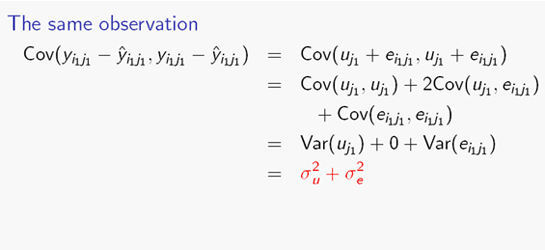So now if we work out the covariance for the same observation, again we're left with the covariance between the error terms, after we've controlled for the x variables, and we can split it up into these covariances. So this covariance is again the covariance of something with itself, so this just becomes the variance of u_j; this covariance is the covariance of a level two error term with a level one error term, so we assumed that's zero; and this covariance is between the same level one error term, so again that becomes a variance, the variance of e_ij; so we're left with the variance of u_j plus the variance of e_ij, and we defined the variance of u_j to be sigma squared_u, we defined the variance of e_ij to be sigma squared_e, so we end up with sigma squared_u plus sigma squared_e so the diagonal terms of this matrix [Matrix 2]are just sigma squared_u plus sigma squared_e.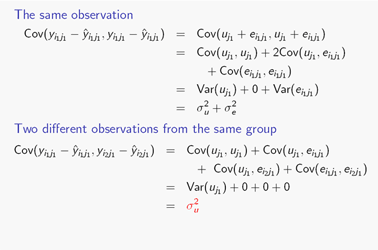So now what about two different observations from the same group? Well, again we can split up the covariance into these covariances. So here we have the covariance of a level two error term with a level one error term, that's zero, by assumption; and again, a level two error term with a level one error term, we assumed that was zero; and now this one is the covariance of two different level one error terms, that's the covariance of the error terms for two different observations in the same group, and we also assumed that was zero. So we're just left with this covariance, which is the covariance of a level two error term with itself, so that's the variance of u_j, and we defined the variance of u_j to be sigma squared_u, so we're left with sigma squared_u.

[Matrix 2]

So the covariance for two different pupils from the same school, that's the off-diagonal terms in the yellow blocks, is just sigma squared_u.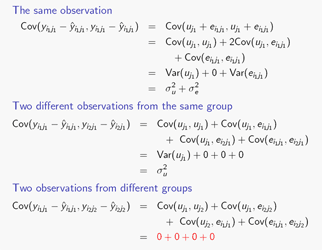And finally for two observations from different groups, again we can split up the covariance. So now this is the covariance between two different level two error terms, we assumed that was zero; this is the covariance between a level two and a level one error term, we assumed that was zero; and again, between a level two and a level one error term, we assumed that was zero; and finally we have the covariance between two different level one error terms, we assumed that was zero. So this time, everything is zero.

### Matrix 2So the covariance between two pupils from different schools is zero, that's the terms outside the yellow blocks. So now let's look at the correlation matrix; again we need to divide by the total variance, and the total variance for a two level random intercept model is the level two plus the level one variance, sigma squared_u plus sigma squared_e, so obviously the diagonal terms are just going to be divided by themselves, they're all going to become 1; the zero terms are obviously going to stay zero; so what happens to the sigma squared_u s? Well, we're going to get sigma squared_u over sigma squared_u plus sigma squared_e, and if you saw the audio recording on measuring dependency, you'll recall that that's just the formula for rhoso this is what we end up with for the correlation matrix.

So now we can see that for this model, we have allowed for the dependency. For two different pupils from different schools, the correlation is zero: they are unrelated, but for two different pupils from the same school, we have a correlation of rho: they are related. And this actually also explains why rho is also called the intraclass correlation: because it's the correlation between two different elements from the same class, two different pupils from the same school. And this shows how the multilevel model has actually allowed for the dependency between observations: when we fitted the multilevel model we've actually allowed two different observations from the same group to be correlated. And that's good because that's what we set out to do.